Anzeige

# Mech 0036 exam 12 13 with answers (revision)

27. Jun 2015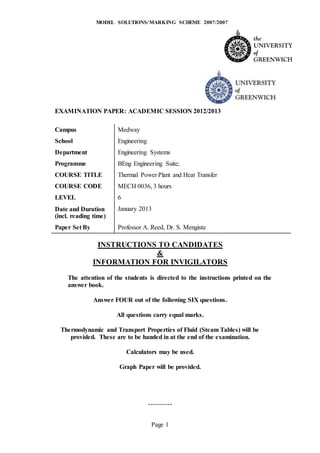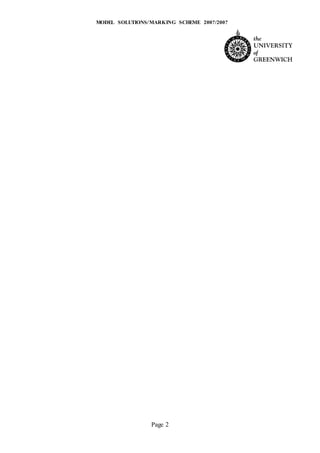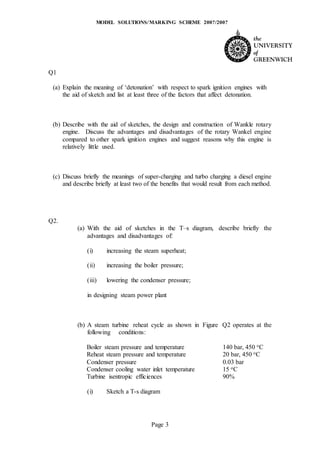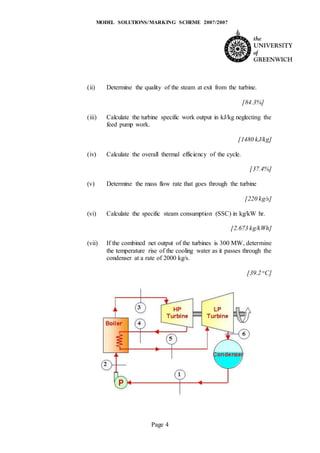Anzeige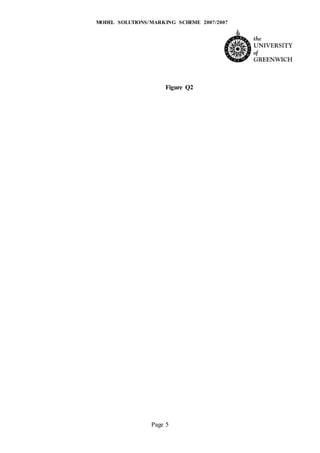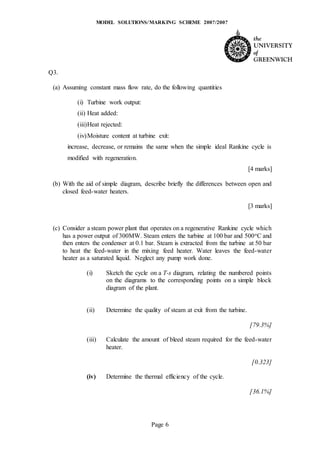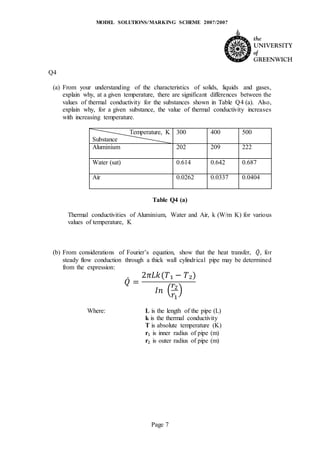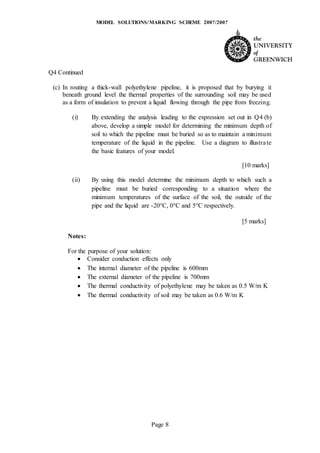Anzeige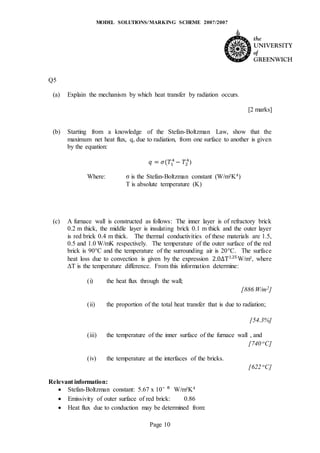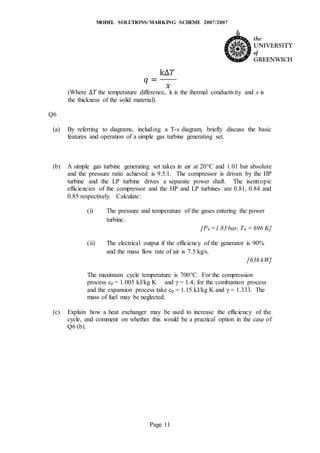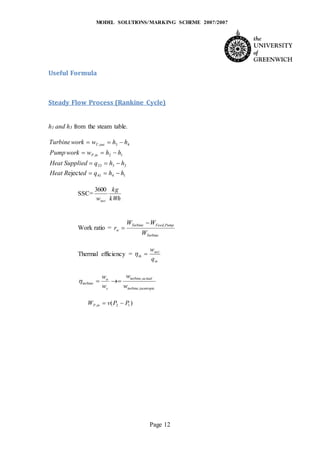Nächste SlideShare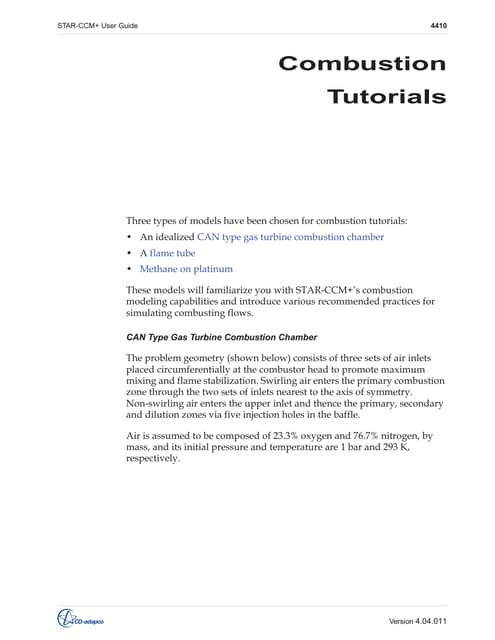Combustion tutorial ( Eddy Break up Model) , CFD
1 von 12
Anzeige

### Mech 0036 exam 12 13 with answers (revision)

1. MODEL SOLUTIONS/MARKING SCHEME 200?/200? Page 1 EXAMINATION PAPER: ACADEMIC SESSION 2012/2013 Campus Medway School Engineering Department Engineering Systems Programme BEng Engineering Suite; COURSE TITLE Thermal Power Plant and Heat Transfer COURSE CODE MECH 0036, 3 hours LEVEL 6 Date and Duration (incl. reading time) January 2013 Paper Set By Professor A. Reed, Dr. S. Mengistu INSTRUCTIONS TO CANDIDATES & INFORMATION FOR INVIGILATORS The attention of the students is directed to the instructions printed on the answer book. Answer FOUR out of the following SIX questions. All questions carry equal marks. Thermodynamic and Transport Properties of Fluid (Steam Tables) will be provided. These are to be handed in at the end of the examination. Calculators may be used. Graph Paper will be provided. ----------
2. MODEL SOLUTIONS/MARKING SCHEME 200?/200? Page 2
3. MODEL SOLUTIONS/MARKING SCHEME 200?/200? Page 3 Q1 (a) Explain the meaning of ‘detonation’ with respect to spark ignition engines with the aid of sketch and list at least three of the factors that affect detonation. (b) Describe with the aid of sketches, the design and construction of Wankle rotary engine. Discuss the advantages and disadvantages of the rotary Wankel engine compared to other spark ignition engines and suggest reasons why this engine is relatively little used. (c) Discuss briefly the meanings of super-charging and turbo charging a diesel engine and describe briefly at least two of the benefits that would result from each method. Q2. (a) With the aid of sketches in the T–s diagram, describe briefly the advantages and disadvantages of: (i) increasing the steam superheat; (ii) increasing the boiler pressure; (iii) lowering the condenser pressure; in designing steam power plant (b) A steam turbine reheat cycle as shown in Figure Q2 operates at the following conditions: Boiler steam pressure and temperature 140 bar, 450 oC Reheat steam pressure and temperature 20 bar, 450 oC Condenser pressure 0.03 bar Condenser cooling water inlet temperature 15 oC Turbine isentropic efficiences 90% (i) Sketch a T-s diagram
4. MODEL SOLUTIONS/MARKING SCHEME 200?/200? Page 4 (ii) Determine the quality of the steam at exit from the turbine. [84.3%] (iii) Calculate the turbine specific work output in kJ/kg neglecting the feed pump work. [1480 kJ/kg] (iv) Calculate the overall thermal efficiency of the cycle. [37.4%] (v) Determine the mass flow rate that goes through the turbine [220 kg/s] (vi) Calculate the specific steam consumption (SSC) in kg/kW hr. [2.673 kg/kWh] (vii) If the combined net output of the turbines is 300 MW, determine the temperature rise of the cooling water as it passes through the condenser at a rate of 2000 kg/s. [39.2 oC]
5. MODEL SOLUTIONS/MARKING SCHEME 200?/200? Page 5 Figure Q2
6. MODEL SOLUTIONS/MARKING SCHEME 200?/200? Page 6 Q3. (a) Assuming constant mass flow rate, do the following quantities (i) Turbine work output: (ii) Heat added: (iii)Heat rejected: (iv)Moisture content at turbine exit: increase, decrease, or remains the same when the simple ideal Rankine cycle is modified with regeneration. [4 marks] (b) With the aid of simple diagram, describe briefly the differences between open and closed feed-water heaters. [3 marks] (c) Consider a steam power plant that operates on a regenerative Rankine cycle which has a power output of 300MW. Steam enters the turbine at 100 bar and 500oC and then enters the condenser at 0.1 bar. Steam is extracted from the turbine at 50 bar to heat the feed-water in the mixing feed heater. Water leaves the feed-water heater as a saturated liquid. Neglect any pump work done. (i) Sketch the cycle on a T-s diagram, relating the numbered points on the diagrams to the corresponding points on a simple block diagram of the plant. (ii) Determine the quality of steam at exit from the turbine. [79.3%] (iii) Calculate the amount of bleed steam required for the feed-water heater. [0.323] (iv) Determine the thermal efficiency of the cycle. [36.1%]
7. MODEL SOLUTIONS/MARKING SCHEME 200?/200? Page 7 Q4 (a) From your understanding of the characteristics of solids, liquids and gases, explain why, at a given temperature, there are significant differences between the values of thermal conductivity for the substances shown in Table Q4 (a). Also, explain why, for a given substance, the value of thermal conductivity increases with increasing temperature. Temperature, K Substance 300 400 500 Aluminium 202 209 222 Water (sat) 0.614 0.642 0.687 Air 0.0262 0.0337 0.0404 Table Q4 (a) Thermal conductivities of Aluminium, Water and Air, k (W/m K) for various values of temperature, K (b) From considerations of Fourier’s equation, show that the heat transfer, 𝑄̇, for steady flow conduction through a thick wall cylindrical pipe may be determined from the expression: 𝑄̇ = 2𝜋𝐿𝑘(𝑇₁ − 𝑇₂) 𝐼𝑛 ( 𝑟2 𝑟1 ) Where: L is the length of the pipe (L) k is the thermal conductivity T is absolute temperature (K) r₁ is inner radius of pipe (m) r₂ is outer radius of pipe (m)
8. MODEL SOLUTIONS/MARKING SCHEME 200?/200? Page 8 Q4 Continued (c) In routing a thick-wall polyethylene pipeline, it is proposed that by burying it beneath ground level the thermal properties of the surrounding soil may be used as a form of insulation to prevent a liquid flowing through the pipe from freezing. (i) By extending the analysis leading to the expression set out in Q4 (b) above, develop a simple model for determining the minimum depth of soil to which the pipeline must be buried so as to maintain a minimum temperature of the liquid in the pipeline. Use a diagram to illustrate the basic features of your model. [10 marks] (ii) By using this model determine the minimum depth to which such a pipeline must be buried corresponding to a situation where the minimum temperatures of the surface of the soil, the outside of the pipe and the liquid are -20°C, 0°C and 5°C respectively. [5 marks] Notes: For the purpose of your solution:  Consider conduction effects only  The internal diameter of the pipeline is 600mm  The external diameter of the pipeline is 700mm  The thermal conductivity of polyethylene may be taken as 0.5 W/m K  The thermal conductivity of soil may be taken as 0.6 W/m K
9. MODEL SOLUTIONS/MARKING SCHEME 200?/200? Page 9 Solution for Q4 as it is not covered in our lecture Part (c ) Suggested model is based on t3 = -20 oC Where minimum depth required: d = r3 – r2 t1 = 5 oC (liquid temperature) t2 = 0 oC (outer pipe temperature) t3 = -20 oC (Ground temperature) this model requires that for steady state condition 𝑄̇ = 2𝜋𝐿𝐾12(𝑡1−𝑡2) ln ( 𝑟2 𝑟1 ) = 2𝜋𝐿𝐾23(𝑡2−𝑡3) ln ( 𝑟3 𝑟2 ) Therefore ln ( 𝑟3 𝑟2 ) = 𝐾23 (𝑡2−𝑡3) 𝐾12(𝑡1−𝑡2) × ln ( 𝑟2 𝑟1 ) ln ( 𝑟3 𝑟2 ) = 0.6 × (0 − (−20)) 0.5 × (5 − 0) × ln ( 0.350 0.3 ) ln ( 𝑟3 𝑟2 ) = 0.7399 𝑟3 = 𝑒0.7399 × 0.350 = 0.733𝑚 So the minimum depth required is d = r3 – r2 = 0.733 – 0.35 =0.38 m
10. MODEL SOLUTIONS/MARKING SCHEME 200?/200? Page 10 Q5 (a) Explain the mechanism by which heat transfer by radiation occurs. [2 marks] (b) Starting from a knowledge of the Stefan-Boltzman Law, show that the maximum net heat flux, q, due to radiation, from one surface to another is given by the equation: 𝑞 = 𝜎(𝑇1 4 − 𝑇2 4 ) Where: σ is the Stefan-Boltzman constant (W/m²K4) T is absolute temperature (K) (c) A furnace wall is constructed as follows: The inner layer is of refractory brick 0.2 m thick, the middle layer is insulating brick 0.1 m thick and the outer layer is red brick 0.4 m thick. The thermal conductivities of these materials are 1.5, 0.5 and 1.0 W/mK respectively. The temperature of the outer surface of the red brick is 90°C and the temperature of the surrounding air is 20°C. The surface heat loss due to convection is given by the expression 2.0∆T1.25 W/m², where ΔT is the temperature difference. From this information determine: (i) the heat flux through the wall; [886 W/m2] (ii) the proportion of the total heat transfer that is due to radiation; [54.3%] (iii) the temperature of the inner surface of the furnace wall , and [740 oC] (iv) the temperature at the interfaces of the bricks. [622 oC] Relevant information:  Stefan-Boltzman constant: 5.67 x 10⁻ ⁸ W/m²K4  Emissivity of outer surface of red brick: 0.86  Heat flux due to conduction may be determined from:
11. MODEL SOLUTIONS/MARKING SCHEME 200?/200? Page 11 𝑞 = kΔ𝑇 𝑥 (Where Δ𝑇 the temperature difference, k is the thermal conductivity and x is the thickness of the solid material). Q6 (a) By referring to diagrams, including a T-s diagram, briefly discuss the basic features and operation of a simple gas turbine generating set. (b) A simple gas turbine generating set takes in air at 20°C and 1.01 bar absolute and the pressure ratio achieved is 9.5:1. The compressor is driven by the HP turbine and the LP turbine drives a separate power shaft. The isentropic efficiencies of the compressor and the HP and LP turbines are 0.81, 0.84 and 0.85 respectively. Calculate: (i) The pressure and temperature of the gases entering the power turbine. [P4 =1.83 bar, T4 = 696 K] (ii) The electrical output if the efficiency of the generator is 90% and the mass flow rate of air is 7.5 kg/s. [636 kW] The maximum cycle temperature is 700°C. For the compression process cp = 1.005 kJ/kg K and γ = 1.4; for the combustion process and the expansion process take cp = 1.15 kJ/kg K and γ = 1.333. The mass of fuel may be neglected. (c) Explain how a heat exchanger may be used to increase the efficiency of the cycle, and comment on whether this would be a practical option in the case of Q6 (b).
12. MODEL SOLUTIONS/MARKING SCHEME 200?/200? Page 12 Useful Formula Steady Flow Process (Rankine Cycle) h1 and h3 from the steam table. 1441 2323 12, 43, eject hhqedRHeat hhqSuppliedHeat hhwworkPump hhwworkTurbine inP outT     SSC= kWh kg wnet 3600 Work ratio = Turbine PumpFeedTurbine w W WW r ,  Thermal efficiency = in net th q w  isentropicturbine actualturbine s a turbine w w w w , ,  )( 12, PPvW inP 
Anzeige